Subject:
Ratios and Proportions
Material Type:
Lesson Plan
Level:
Middle School
7
Provider:
Pearson
Tags:
7th Grade Mathematics, Distributive Property, Proportionality
Language:
English
Media Formats:
Text/HTML

# Percent Decrease Problems## Overview

Students represent and solve percent decrease problems.

# Key Concepts

When there is a percent decrease between a starting amount and a final amount, the relationship can be represented by an equation of the form y = kx where y is the final amount, x is the starting amount, and k is the constant of proportionality, which is equal to 1 minus the percent change, p, represented as a decimal: k = 1 – p, so y = (1 – p)x.

The constant of proportionality k has the value it does—a number less than 1—because of the way the distributive property can be used to simplify the expression for the starting amount decreased by a percent of the starting amount: xx(p) = x(1 – p).

# Goals and Learning Objectives

• Determine the unknown amount—either the starting amount, the percent change, or the final amount—in a percent decrease situation when given the other two amounts.
• Make a table to represent a percent decrease problem.
• Write and solve an equation to represent a percent decrease problem.

# Lesson Guide

• Have students read the prompt and questions.
• Have pairs of students share ideas about situations involving percent decrease.

# Mathematics

Students are asked to reflect on the percent increase problems they worked on in previous lessons and to think about situations involving percent decrease instead.

ELL: Encourage students to verbalize their explanations. Allow students to speak in small groups to gain confidence.

ELL: Note that the terms like percent change may still need some explanation. ELLs may not understand the context of the term and therefore not understand the goal of the session. Other terms that may present problems in this session include:

• Starting amount
• Final amount
• Constant of proportionality
• Distributive property
• Decimal

# Percent Decrease Situations

Think about the percent increase problems you worked on.

• What types of situations do you think would involve percent decrease? Can you think of an example?

# Lesson Guide

Discuss the Math Mission. Students will represent and solve percent decrease problems.

SWD: Students with disabilities may not as readily connect new information to previously learned skills and concept. Activate background knowledge about percent increase to promote connections between this and the new topic being discussed here.

## Opening

Represent and solve percent decrease problems.

# Lesson Guide

Have students work in pairs on all problems and the presentation.

# Mathematical Practices

Mathematical Practice 7: Look for and make use of structure.

Look for students who are using the structure of the relationships among quantities to reason about solutions that make sense.

Mathematical Practice 2: Reason abstractly and quantitatively.

Identify students who reason whether solutions make sense given their meaning in the situation and given the values in the situation.

# Interventions

[common error] Student sets up an incorrect equation.

• Is the unknown amount the starting amount, final amount, or percent change? Where is the unknown amount in your equation?
• Does your solution make sense in this situation? If not, check the structure of your equation. Does it make sense?

[common error] Student sets up a correct equation, but solves it incorrectly.

• Does your solution make sense in this situation?
• Should your solution be greater or less than the final amount [starting amount]?

Student completes problems efficiently and correctly.

• In this lesson’s problems, you wrote equations that expressed the final amount in terms of the starting amount. Can you write a new equation for each situation that expresses the starting amount in terms of the final amount?

SWD: Students with disabilities may have difficulty with cooperative learning tasks. Support students who have trouble resolving conflicts or disagreements by coaching them to recognize the importance of considering alternate perspectives. Also, provide suggestions as to how students can navigate differences of opinion in learning situations.

SWD: Some students with disabilities can get frustrated when working with decimals. If so, provide them access to a calculator so they can focus on learning and practicing the more complex, conceptual elements of this task.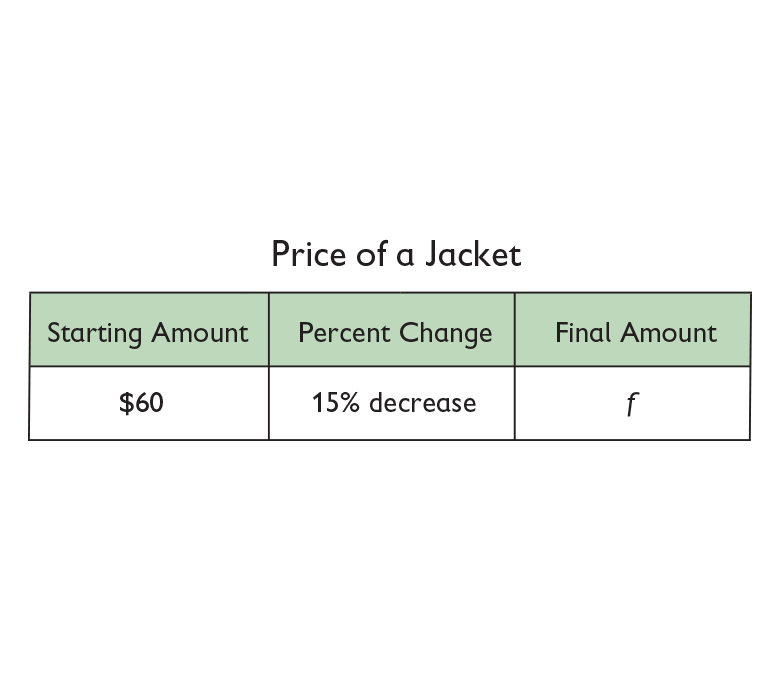• Equation:

f = 60 – (0.15)(60)

= (1 – 0.15)(60)

= 0.85(60)

• Solution:

f = 0.85(60)

f = 51

The new price of the jacket is $51.00. ## Work Time # Price of a Jacket A store manager reduces the price of a jacket by 15%. The jacket originally cost$60. What is the new price?

• Copy and complete the table.
• Write an equation.
• Write the solution as a complete sentence.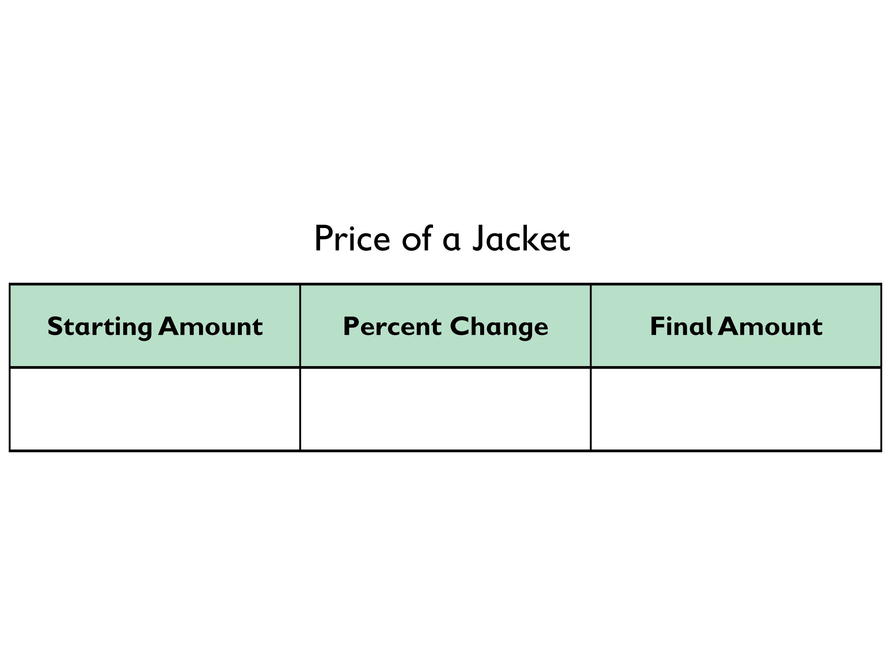## Hint:

• Remember that the price has been decreased—not increased. What should you do differently in setting up the equation as compared to the way you set up an equation to represent percent increase?
• Is the unknown amount the starting amount, the final amount, or the percent change? How can you represent the unknown amount in your equation?

# Lesson Guide

Have students work in pairs on all problems and the presentation.

# Interventions

[common error] Student sets up an incorrect equation.

• Is the unknown amount the starting amount, final amount, or percent change? Where is the unknown amount in your equation?
• Does your solution make sense in this situation? If not, check the structure of your equation. Does it make sense?

[common error] Student sets up a correct equation, but solves it incorrectly.

• Does your solution make sense in this situation?
• Should your solution be greater or less than the final amount [starting amount]?

Student completes problems efficiently and correctly.

• In this lesson’s problems, you wrote equations that expressed the final amount in terms of the starting amount. Can you write a new equation for each situation that expresses the starting amount in terms of the final amount?

•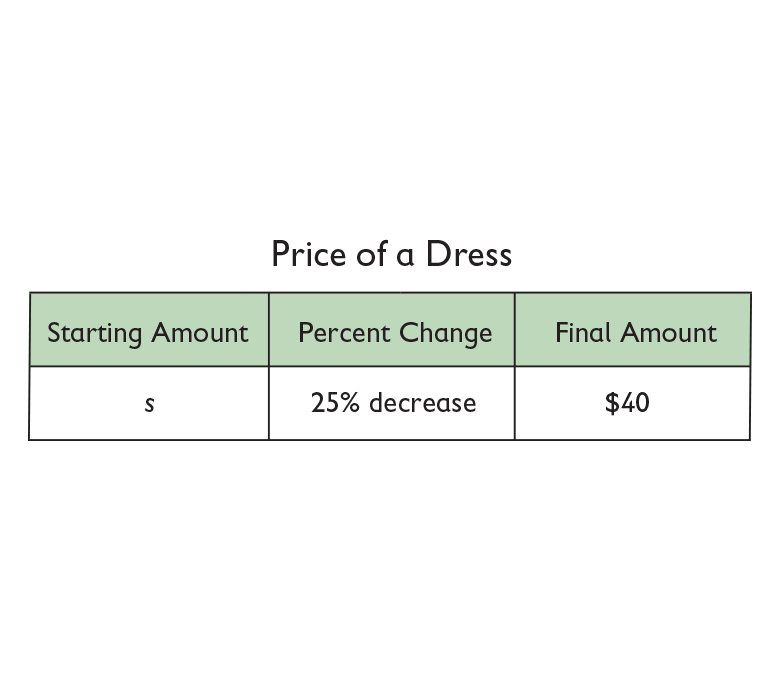• Equation:

40 = s – 0.2s

= (1 – 0.2)s

= 0.8s

• Solution:

40 = 0.8s

$\frac{40}{0.8}=\frac{0.8s}{0.8}$

50 = s

Before the sale, the price of the dress was $50.00. ## Work Time # Price of a Dress A sign on a dress in a store window says: “20% off! Sale price$40!” What was the price of the dress before the sale?

• Copy and complete the table.
• Write an equation.
• Write the solution as a complete sentence.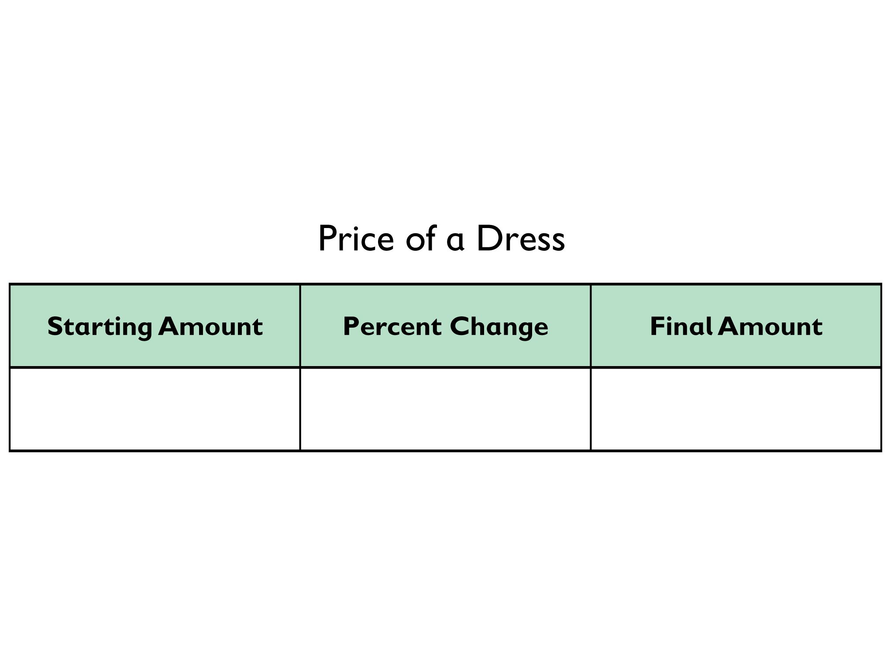## Hint:

• Remember that the price has been decreased—not increased. What should you do differently in setting up the equation as compared to the way you set up an equation to represent percent increase?
• Is the unknown amount the starting amount, the final amount, or the percent change? How can you represent the unknown amount in your equation?

# Lesson Guide

Have students work in pairs on all problems and the presentation.

# Interventions

[common error] Student sets up an incorrect equation.

• Does your solution make sense in this situation? If not, check the structure of your equation. Does it make sense?
• In the bus ticket problem, what should be multiplied by the percent change in your equation?

[common error] Student sets up a correct equation, but solves it incorrectly.

• Does your solution make sense in this situation?
• In the bus ticket problem, should your solution be greater or less than 100%?
• In the bus ticket problem, look at the starting amount and final amount, and make an estimate of the percent decrease between them. Is your solution close to your estimate?

Student completes problems efficiently and correctly.

• In this lesson’s problems, you wrote equations that expressed the final amount in terms of the starting amount. Can you write a new equation for each situation that expresses the starting amount in terms of the final amount?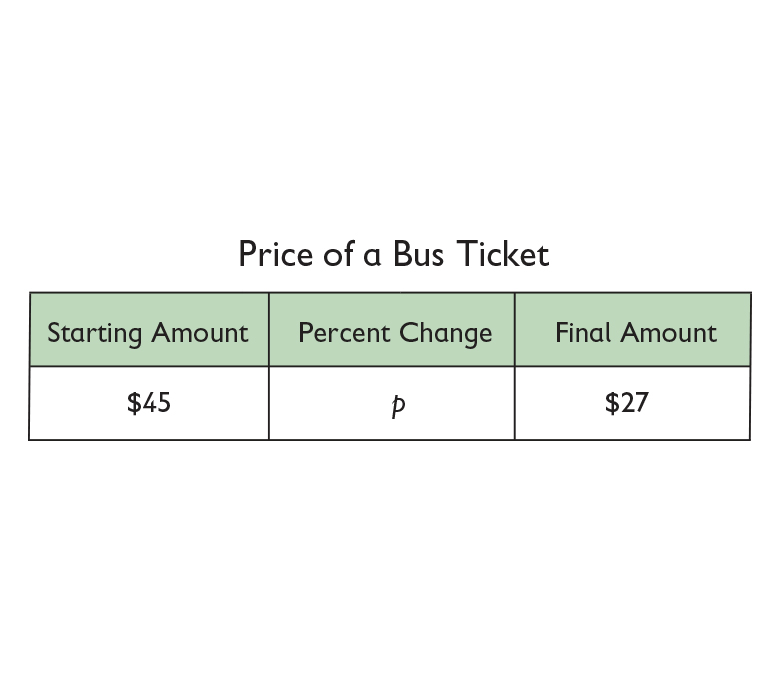• Equation:

27 = 45 − 45p

27 = 45(1 − p)

$p=1-\frac{27}{45}$

• Solution:

$p=1-\frac{27}{45}$

p = 1 – 0.6

p = 0.4

The cost of the bus ticket decreased 40%.

# Price of a Bus Ticket

A bus ticket normally costs $45. As a special offer, the bus company reduces the price to$27. What is the percent change?

• Copy and complete the table.
• Write an equation.
• Write the solution as a complete sentence.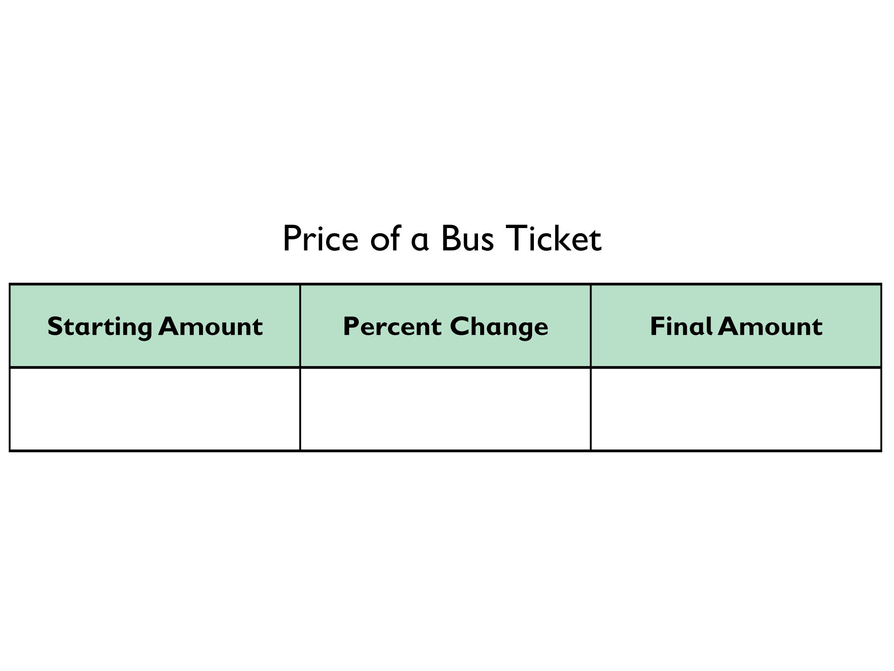## Hint:

• Remember that the price has been decreased—not increased. What should you do differently in setting up the equation as compared to the way you set up an equation to represent percent increase?
• Is the unknown amount the starting amount, the final amount, or the percent change? How can you represent the unknown amount in your equation?

# Preparing for Ways of Thinking

Look and listen for students who:

• Understand that each relationship between starting and final amounts is a proportional relationship.
• Understand why each constant of proportionality has the value it does.
• Use the distributive property to write or simplify equations in each problem.
• Understand the parallel structure between percent increase and percent decrease situations.

# Challenge Problem

Answers will vary. Possible answer: The distributive property is used in the same way in percent increase and percent decrease problems, but the result is different. In percent increase problems, the percent (as a decimal number) is added to 1, which represents the initial amount (one whole, to which a percent of that whole is added), so the result is a multiplier that is greater than 1. In percent decrease problems, the percent (as a decimal number) is subtracted from 1, which represents the initial amount, so the result is a multiplier that is less than 1.

# Prepare a Presentation

Prepare a presentation in which you show and explain each part of your work for all three of the percent decrease problems.

# Challenge Problem

Explain how you used the distributive property to solve percent increase problems. Can you use the distributive property in this way to solve percent decrease problems?

# Mathematics

Bring out any variation in approaches to writing and solving equations in the three problems. Use the variation in approaches to highlight the use of the distributive property to simplify expressions.

Have students reflect on their work on the bus ticket problem by asking:

• How did you know that the percent change was a decrease and not an increase?
• Explain how you know from reasoning about the values given for the situation.
• Explain how you know from the mathematical structure of the situation.

Have students present any work they did on the Challenge Problem.

# Mathematical Practices

Mathematical Practice 7: Look for and make use of structure.

Help students recognize the fact that they are using the structure of the mathematics in order to make sense of what is going on.

# Ways of Thinking: Make Connections

Take notes about your classmates’ approaches and explanations for solving the three percent decrease problems.

## Hint:

• Is the unknown amount the starting amount, the final amount, or the percent change?
• Where is the unknown amount in your equation?
• Why does your solution make sense in this situation?
• Should the solution be greater than or less than the given amount?
• Should the solution be greater than or less than 100%?
• How did you use the mathematical structure of the situation to help you solve the problem?
• How is solving a percent decrease problem similar to solving a percent increase problem? How is it different?

# Lesson Guide

• Have pairs quietly read and then discuss how to calculate a percent decrease.
• As student pairs work together, make a note to clarify any misunderstandings in the class discussion.
• After a few minutes, discuss the summary as a class. Be sure to include the following points:
• There are many different kinds of situations involving a percent decrease.
• No matter what the context, a table can help you organize the information so that you can solve the problem.

# Summary of the Math: Percent Decrease

• A percent can describe a change in a value that decreases. Such a percent is called a percent decrease.
• If an amount decreases by x%, you can multiply that amount by (100% − x%) to determine the final amount.
• For example, if the percent decrease is 5% and the original amount is m, then 100 − 5 = 95% = 0.95
0.95m = the final amount

## Hint:

Can you:

• Explain how to calculate a percent decrease?
• Give examples of situations that involve percent decrease?
• Describe how to use a table to organize the information in a percent decrease problem?